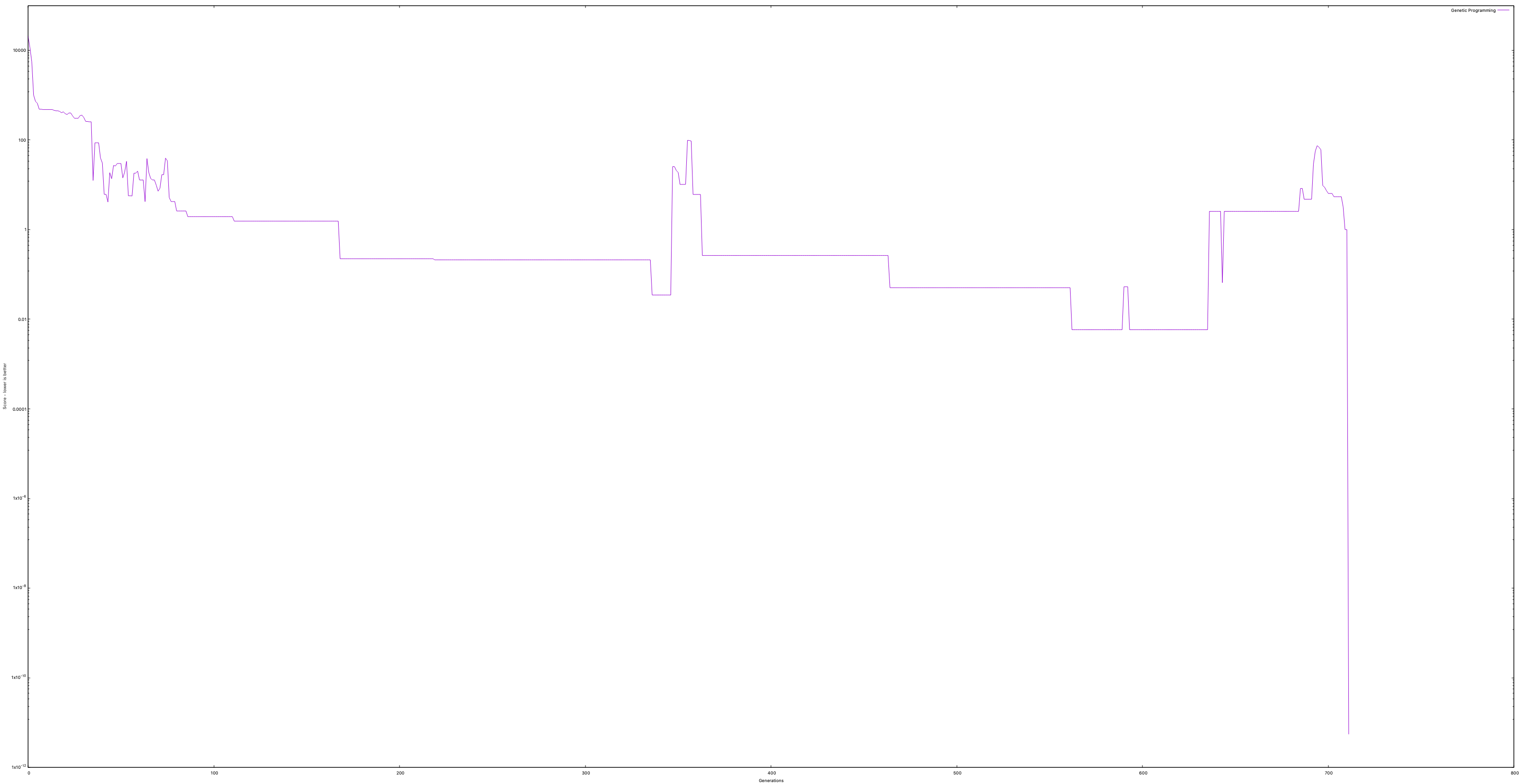I found a copy of Programming Collective Intelligence while dusting off the bookshelf. The book is now 11 years old.

Genetic Programming is introduced in chapter eleven and I’ve decided to replicate the example using Kotlin instead of Python.

The source code can be found here.

The idea is to simulate biological evolution to derive a program that is best fitted to solve our problem.

We start by creating a small language. A set of programs written in our language defines a population that through evolution will yield the program that when evaluated will give the best answer to solve our problem.

The evolution process uses breeding and mutation to create new populations. The programs in a population are scored and ranked on each generation. New populations are then created based off the best programs from the previous population.

We keep producing new generations of programs until we either reach a perfect solution or the evolution hits a hard limit. In our implementation the hard limit is defined by a max number of generations that can be created.

The example in chapter eleven tries to derive an expression that is close to the hidden function

``````fun hiddenFunction(x: Double, y: Double): Double =
x.pow(2) + 2 * y + 3 * x + 5
``````

Notice that different from the book example I’ve used a Double instead of an Integer. I thought it would be more interesting to find an expression that yields results closer to the hidden function and unlikely to find a perfect expression.

Here’s how we’ve defined our expression language. Again, the source code is available on github.

The only method we care about is `evaluate`

``````sealed class Expr {
abstract fun evaluate(ctx: Context): Double
}

data class Const(val v: Double) : Expr() {
override fun evaluate(ctx: Context): Double = v
}

data class Param(val name: String) : Expr() {
override fun evaluate(ctx: Context): Double =
ctx.getOrElse(name, { throw RuntimeException("Mal-formed Context") })
}

data class Add(val e1: Expr, val e2: Expr) : Expr() {
override fun evaluate(ctx: Context): Double =
e1.evaluate(ctx) + e2.evaluate(ctx)
}

data class Sub(val e1: Expr, val e2: Expr) : Expr() {
override fun evaluate(ctx: Context): Double =
e1.evaluate(ctx) - e2.evaluate(ctx)
}

data class Mul(val e1: Expr, val e2: Expr) : Expr() {
override fun evaluate(ctx: Context): Double =
e1.evaluate(ctx) * e2.evaluate(ctx)
}

data class If(val cond: Expr, val exprTrue: Expr, val exprFalse: Expr) : Expr() {
override fun evaluate(ctx: Context): Double =
e1.evaluate(ctx) * e2.evaluate(ctx)
}

data class Gt(val e1: Expr, val e2: Expr) : Expr() {
override fun evaluate(ctx: Context): Double =
if (e1.evaluate(ctx) > e2.evaluate(ctx)) 1.0 else 0.0
}
``````

The Context is used to load user variables, or as we call it, Param.

``````typealias Context = Map<String, Double>
``````

After we’ve defined our expression language the creation of a random program is trivial. The breeding and mutation functions used in the evolution process are simple tree manipulations.

I’ve recorded a sample run on my machine and capture the score of each expression. Notice how over time they approach zero which is the perfect solution.Another interesting plot is a comparison between the hidden function and the expression built by evolution.The x and y axes represent the input of our function and the z axis the expression evaluation.